# RS Aggarwal Class 7 Solutions Chapter 4 Rational Numbers Ex 4A

In this chapter, we provide RS Aggarwal Solutions for Class 7 Chapter 4 Rational Numbers Ex 4A for English medium students, Which will very helpful for every student in their exams. Students can download the latest RS Aggarwal Solutions for Class 7 Chapter 4 Rational Numbers Ex 4A Maths pdf, free RS Aggarwal Solutions Class 7 Chapter 4 Rational Numbers Ex 4A Maths book pdf download. Now you will get step by step solution to each question.

### RS Aggarwal Solutions for Class 7 Chapter 4 Rational Numbers Ex 4A Download PDF

Question 1.
Solution:
(i) Rational numbers: The numbers of the form pq where p and q are integers and q ≠ 0, are called rational numbers.(iv) Yes, there is one rational number (0) which is neither positive nor negative.

Question 2.
Solution:(viii) 01 are all rational number but 10 and 00 are not rational number as their denominator is zero.

Question 3.
Solution:
(i) Numerator = 8, denominator =19
(ii) Numerator = 5, denominator = – 8
(iii) Numerator =-13, denominator =15
(iv) Numerator = – 8, denominator = -11
(v) Numerator = 9, denominator = 1

Question 4.
Solution:Question 5.
Solution:
According to the definition, a rational number is positive if both of numerator and denominator have same signs. Therefore
(iii), (iv) and (vi) 8 are positive rational numbers.

Question 6.
Solution:
According to the definition, a rational number is negative if numerator and denominator have opposite sign. Therefore.
(iii), (iv), (v), (vi) are all negative rational numbers.

Question 7.
Solution:
Equivalent rational numbers of each are given below: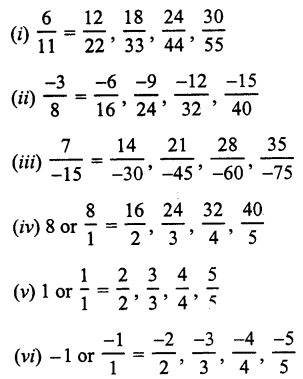Question 8.
Solution:Question 9.
Solution:Question 10.
Solution:Question 11.
Solution: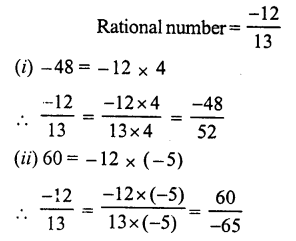Question 12.
Solution:Question 13.
Solution: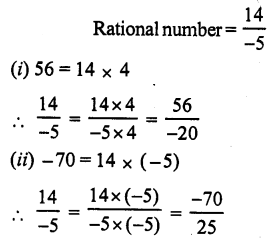Question 14.
Solution: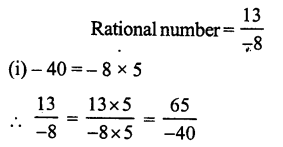Question 15.
Solution:Question 16.
Solution:Question 17.
Solution: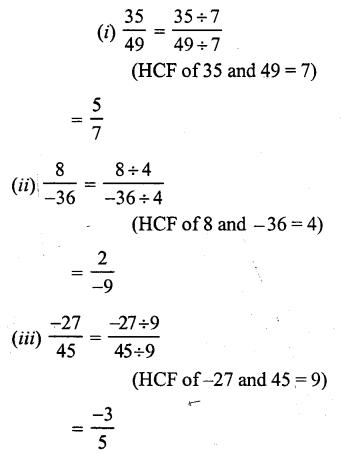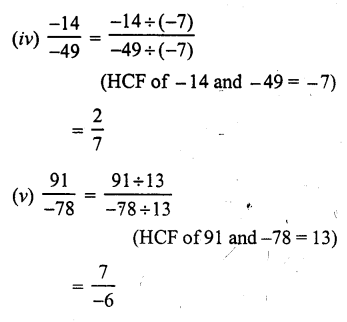Question 18.
Solution:Question 19.
Solution: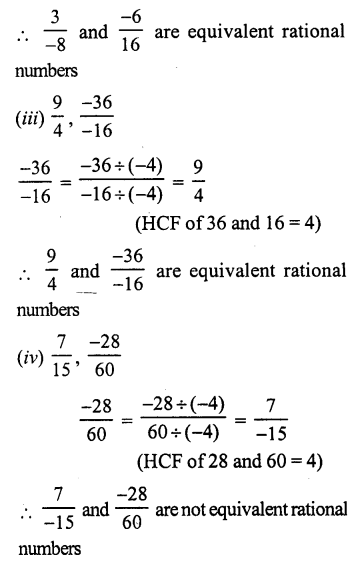Question 20.
Solution: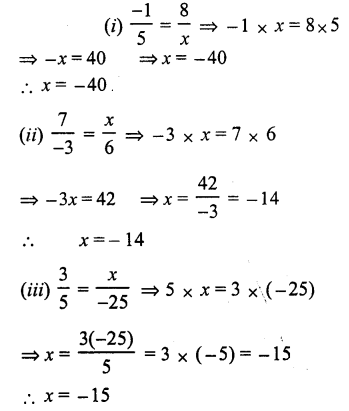Question 21.
Solution:Question 22.
Solution:
(i) False, as there is no end of smallest and largest rational number,
(ii) True.
(iii) False, as zero is a rational number but the division of zero is meaningless.
(iv) True.
(v) False, every rational is not a fraction
In a fraction, numerator and denominators is a whole number but the denominator can’t be zero

All Chapter RS Aggarwal Solutions For Class 7 Maths

—————————————————————————–

All Subject NCERT Exemplar Problems Solutions For Class 7

All Subject NCERT Solutions For Class 7

*************************************************

I think you got complete solutions for this chapter. If You have any queries regarding this chapter, please comment on the below section our subject teacher will answer you. We tried our best to give complete solutions so you got good marks in your exam.

If these solutions have helped you, you can also share rsaggarwalsolutions.in to your friends.